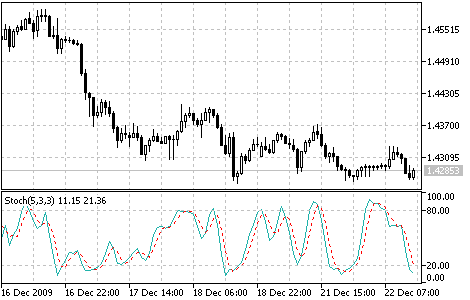Interesting script?
So post a link to it -
let others appraise it

You liked the script? Try it in the MetaTrader 5 terminal# Stochastic Oscillator - indicator for MetaTrader 5

Views:
12876
Rating:
Published:
2010.01.26 11:24
Updated:
2016.11.22 07:32

The Stochastic Oscillator technical indicator compares where a security’s price closed relative to its price range over a given time period.

The Stochastic Oscillator is displayed as two lines. The main line is called %K. The second line, called %D, is a Moving Average of %K. The %K line is usually displayed as a solid line and the %D line is usually displayed as a dotted line.

There are several ways to interpret a Stochastic Oscillator. Three popular methods include:

• Buy when the Oscillator (either %K or %D) falls below a specific level (for example, 20) and then rises above that level.Sell when the Oscillator rises above a specific level (for example, 80) and then falls below that level;
• Buy when the %K line rises above the %D line and sell when the %K line falls below the %D line;
• Look for divergences. For instance: where prices are making a series of new highs and the Stochastic Oscillator is failing to surpass its previous highs.Stochastic Oscillator

Calculation:

The Stochastic Oscillator has four variables:

• %K period. This is the number of time periods used in the stochastic calculation;
• %K Slowing Period. This value controls the internal smoothing of %K. A value of 1 is considered a fast stochastic; a value of 3 is considered a slow stochastic;
• %D period. This is the number of time periods used when calculating a moving average of %K;
• %D smoothing method. The method (i.e., Exponential, Simple, Smoothed, or Weighted) that is used to calculate %D.

The formula for %K is:

%K = (CLOSE-LOW(%K))/(HIGH(%K)-LOW(%K))*100

where:

• CLOSE - is today’s closing price;
• LOW(%K) - the lowest low in %K periods;
• HIGH(%K) - the highest high in %K periods.

The %D moving average is calculated according to the formula:

%D = SMA(%K, N)

where:

Translated from Russian by MetaQuotes Software Corp.
Original code: https://www.mql5.com/ru/code/50Standard Deviation (StdDev)

The Standard Deviation (StdDev) measures the market volatility. This indicator charactrizes the scale of price changes relating to the Moving Average.Relative Vigor Index (RVI)

The main point of the Relative Vigor Index Indicator (RVI) is that on the bull market the closing price is, as a rule, higher, than the opening price.Ultimate Oscillator

The Ultimate Oscillator was developed by Larry Williams. It uses the averaging of three oscillators with different periods.Volumes

The Volumes indicator shows volumes with different colors depending on the volume changes.